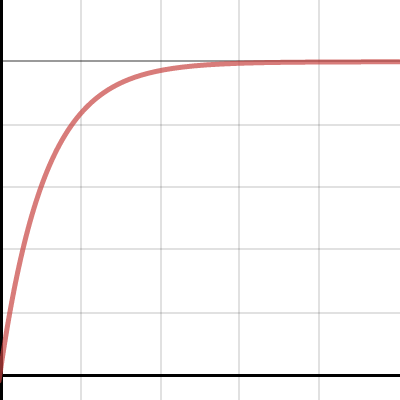Dear Uncle Colin,

I’m looking for the equation of a curve that goes through the points $\left(10, \frac{1}{64}\right)$ and $\left(100, \frac{1}{32} \right)$, as – as $x$ gets large – approaches 1. How do I go about it?

Always Silence Your Mobile Phone Typing Out Tricky Equations

Good advice, ASYMPTOTE! And a great question.

There are infinitely many curves that fit the bill, but the one that jumps out at me is of the form $y = 1 - Ae^{-kx}$. From here, it’s ‘simply’ a case of working out $A$ and $k$.

We have two equations:

$\frac{1}{64} = 1 - Ae^{-10k}$

$\frac{1}{32} = 1 - Ae^{-100k}$

A little rearrangement to make them (slightly) nicer gives:

$\frac{64}{63} = Ae^{10k}$

$\frac{32}{31} = Ae^{100k}$

Dividing these gives

$\frac{32 \times 63}{31 \times 64} = e^{90k}$

With a quick cancel and a logarithm:

$\frac{1}{90}\ln\left(\frac{63}{62}\right) = k$.

We can substitute this back into one of the earlier equations to find $A$:

$\frac{64}{63} = A e^{-\frac{1}{9} \ln\left(\frac{63}{62}\right)}$

Simplify the exponential:

$\frac{64}{63} = A \left(\frac{63}{62}\right)^{-\frac 19}$

$\frac{64}{63} \left(\frac{63}{62}\right)^{\frac 19}= A$